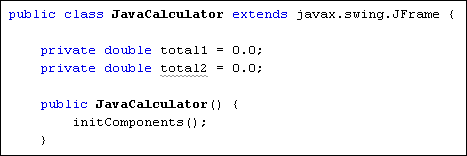Home and Learn: Games Programming Course

# Coding the Equals Button of our Java Calculator

Now that you have some code for your Plus button, we can tackle the equals button.

After the user has chosen a second number, the equals button needs to be clicked. Clicking the equals button will produce the answer to the addition.

To store the output of the calculation, we can set up another field variable. Add the following line to the top of your code:

private double total2 = 0.0;

Your code window should look like this:To get the answer to the calculation, we take whatever is currently stored in total1 and add it to whatever is currently in the text field. Again, though, we need to parse the string from the text field and turn it into a double.

Go back to Design view and double click your equals button. In the code stub that is created, add the following line:

total2 = total1 + Double.parseDouble( txtDisplay.getText( ) );

This line gets the text from the text field and converts the string into a double. The result is then added to total1. The answer is then stored in the total2 variable.

The next thing we need to do is to display the answer to the calculation back into the text field. However, we now have to convert the double back into a string, as text fields hold text and not numbers. If you try to store a double value directly into a text field you'll get errors.

To convert a double into text you can use the toString method of the Double object. Add the following line just below the first one:

txtDisplay.setText( Double.toString( total2) );

The conversion is done between the round brackets of setText. But you could set up a new variable, if you wanted:

String s1 = Double.toString( total2 );
txtDisplay.setText( s1 );

But the result is the same: convert a double to a string.

The final line for the equals button can clear the total1 variable. Once the total1 variable is cleared, a new calculation can be entered. Here's the line to add:

total1 = 0;

The three lines for your equals button should now be these:

total2 = total1 + Double.parseDouble( txtDisplay.getText( ) ) ;
txtDisplay.setText( Double.toString(total2) );
total1 = 0;

In the next part, we'll write code for the Clear button. You'll also get to try a few exercises.

Back to the Java Contents Page

Email us: enquiry at homeandlearn.co.uk